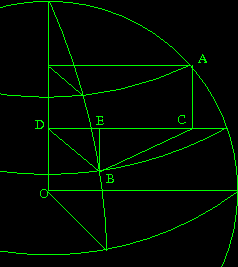## 30-Mar-2007

### Distance between points on a sphere

Filed under: math — jlm @ 15:22

If you try and find out how you compute the distance between points on a sphere, you’ll get a bunch of sites which offer to calculate it for you if you enter the coordinates. If you search harder, you can even get the formula. But no one seems to be offering a derivation. So here you go.

First, some preliminaries. The half angle formulas give us 2 sin² ½ψ = 1-cos ψ. (Easily derivable from cos 2α = cos² α – sin² α.) The straight-line distance (cutting through the inside of the sphere) between two points on a unit sphere with an angle ψ between them is 2 sin ½ψ. (Bisect the triangle formed by the two points and the center of the sphere.)Consider A and B to be our two points we want to get the distance between, with latitudes φA and φB, and longitudes which differ by Θ. We’ll operate mostly on the disk formed by the parallel through B. The point where it intersects the pole is D, and the projection of A onto it is C. Let EB be perpendicular to DC.

The radius of the parallel through A is cos φA = DC; the one through B is cos φB = DB. Angle CDB is Θ, so EB = rB sin Θ and ED = rB cos Θ.
EC = CD – ED = rA – rB cos Θ.
BC² = EC² – EB² = rA² – 2 rA rB cos Θ + rB² cos² Θ + rB² sin² Θ = rA² + rB² – 2 rA rB cos Θ.

AC = |sin φA – sin φB|.
AB² = BC² + AC² = rA² + rB² – 2 rA rB cos Θ + sin² φA + sin² φB – 2 sin φA sin φB
= 2 – 2 rA rB cos Θ – 2 sin φA sin φB.

If ψ is the angle AOB (which is also the along-surface distance between A and B), then
AB² = 4 sin² ½ψ = 2 – 2 cos ψ.
So, 1 – rA rB cos Θ – sin φA sin φB = 1 – cos ψ.
ψ = arccos(cos φA cos φB cos Θ + sin φA sin φB).